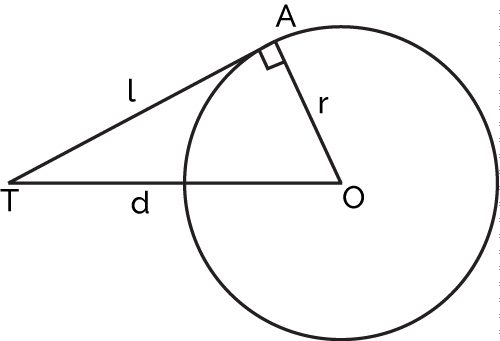# Tangent of a Circle Calculator

Created by Rahul Dhari
Reviewed by Steven Wooding
Last updated: Mar 11, 2022

This tangent of a circle calculator computes the length of the tangent of a circle. A tangent line of a circle is a very versatile property of a circle that will not change even during different transformations, mapping, and scalings. A tangent is very crucial to various geometric constructions and theorems.

A tangent can be used for different applications in the differentials and approximations, strength of materials, motion, distance, and so on. In this article, you'll read more on what is a tangent, and how to find the tangent of a circle, concluding with a discussion on several applications of tangents.

## What is a tangent of a circle?

The definition of the tangent of a circle is given as a line beginning from a point on a circle while being perpendicular to the radius. A tangent of the circle touches, not intersects, the circle at one point only.

Consider a circle with center O and a point A on the circle. The line joining the points O and A be OA. The tangent of a circle from the point A is perpendicular to the line OA. Now, if you draw a line between the point O and a point T on the tangent line.

Note that the tangent of a circle is perpendicular to the radius, i.e., the lines joining the points O, A, and T forms a right-angled triangle. The equation of tangent of a circle is also based on Pythagoras's theorem. The length of the lines OA, AT, and OT can be given by the tangent of a circle formula. Mathematically, this is expressed as:

OA2 + AT2 = OT2

Let the radius of the circle be r, and the length of the tangent be l. The distance d between the point on the tangent T and the center of the circle O can also be written as:

r2 + l2 = d2

In other words, the length of the tangent can be given by the equation of tangent to a circle:

l = √(r2 - d2)Now, consider a circle with center (a, b) having radius r. The equation of circle can be written as:

(x - a)2 + (y - b)2 = r2

Then the equation of tangent with point (x1, y1) is given by the equation:

(x - x1)(x1 - a) + (y - y1)(y1 - b) = 0

## How to find the tangent of a circle?

To find the tangent of a circle at a point:

1. Insert the radius of circle, r.
2. Enter the distance d between the center and point on a tangent.
3. The tangent of a circle calculator will now calculate the length of the tangent l for you.

## Example: Using the tangent of a circle calculator

Now that you know what is a tangent of a circle, let's try to solve a real tangent of a circle problem. Determine the length of the tangent of a circle having a radius of 10 m, and the point on the tangent being 15 m from the center.

To find the length of tangent:

1. Insert the radius of circle, r = 10 m.
2. Enter the distance, d between the center and point on tangent as 15 m.
3. Using the tangent of a circle formula:
l = (d2 - r2)0.5 = (152 - 102)0.5 = 11.18 m

## Applications of the tangent line of a circle

The tangent of a circle is extensively used in various advanced concepts such as:

• Approximations and differential – The slope of the tangent at a point on a curve defined by the function f(x) can be given by the first derivative f'(x).
• Geometry – The combination of various tangents on the circle can be used to construct a tangential polygon. The circle then is called an incircle of the polygon.
• Power transmission – Two circles joined by a pair of mutual tangents can be used to solve the problem of belt length over pulleys. The sum of the length of tangent lines and the arc subtended by them can be used to find the length of the belt.
• Mechanics – The slope of the tangent line on the curve representing the velocity of an object gives the instantaneous velocity of the object.

## FAQ

### Define tangent of a circle?

The tangent of a circle is given by the line from the point on the circle, perpendicular to the radius line.

### How to calculate length of tangent of a circle?

To calculate the length of the tangent:

1. Find the square of the distance between center and point on the tangent.
2. Subtract the square of the radius of the circle from the result of step 1.
3. Obtain the square root of the result from step 2.

### What is the equation of tangent of circle?

The equation of the tangent line of the circle is given by:

(x - x1)(x1 - a) + (y - y1)(y1 - b) = 0

### What is the tangent of circle formula?

The length of tangent of circle, l is given by the equation:

l = √(d2 - r2)
Rahul Dhariin
Length of side OT (d)
in
Length of tangent (l)
in
People also viewed…

### Discriminant

The discriminant calculator is here whenever you need to find the discriminant of any polynomial whose degree is between two and five.

### Significant figures

The significant figures calculator performs operations on sig figs and shows you a step-by-step solution!

### Snowman

The perfect snowman calculator uses math & science rules to help you design the snowman of your dreams!

### Three-dimensional distance

Use the three-dimensional distance calculator to find the distance between two points in space.